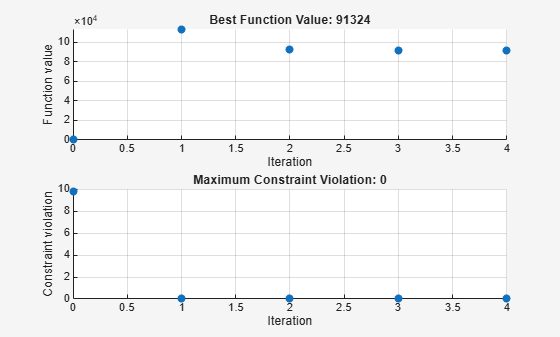## Constrained Minimization Using Pattern Search

This example shows how to minimize an objective function, subject to nonlinear inequality constraints and bounds, using pattern search.

### Constrained Minimization Problem

For this problem, the objective function to minimize is a simple function of a 2-D variable `x`.

` simple_objective(x) = (4 - 2.1*x(1)^2 + x(1)^4/3)*x(1)^2 + x(1)*x(2) + (-4 + 4*x(2)^2)*x(2)^2;`

This function is known as "cam," as described in L.C.W. Dixon and G.P. Szego .

Additionally, the problem has nonlinear constraints and bounds.

``` x(1)*x(2) + x(1) - x(2) + 1.5 <= 0 (nonlinear constraint) 10 - x(1)*x(2) <= 0 (nonlinear constraint) 0 <= x(1) <= 1 (bound) 0 <= x(2) <= 13 (bound) ```

### Code the Objective Function

Create a MATLAB file named `simple_objective.m` containing the following code:

`type simple_objective`
```function y = simple_objective(x) %SIMPLE_OBJECTIVE Objective function for PATTERNSEARCH solver % Copyright 2004 The MathWorks, Inc. x1 = x(1); x2 = x(2); y = (4-2.1.*x1.^2+x1.^4./3).*x1.^2+x1.*x2+(-4+4.*x2.^2).*x2.^2; ```

Solvers such as `patternsearch` accept a single input `x`, where `x` has as many elements as the number of variables in the problem. The objective function computes the scalar value of the objective function and returns it in its single output argument `y`.

### Coding the Constraint Function

Create a MATLAB file named `simple_constraint.m` containing the following code:

`type simple_constraint`
```function [c, ceq] = simple_constraint(x) %SIMPLE_CONSTRAINT Nonlinear inequality constraints. % Copyright 2005-2007 The MathWorks, Inc. c = [1.5 + x(1)*x(2) + x(1) - x(2); -x(1)*x(2) + 10]; % No nonlinear equality constraints: ceq = []; ```

The constraint function computes the values of all the inequality and equality constraints and returns the vectors `c` and `ceq`, respectively. The value of `c` represents nonlinear inequality constraints that the solver attempts to make less than or equal to zero. The value of `ceq` represents nonlinear equality constraints that the solver attempts to make equal to zero. This example has no nonlinear equality constraints, so `ceq = []`. For details, see Nonlinear Constraints (Optimization Toolbox).

### Minimize Using `patternsearch`

Specify the objective function as a function handle.

`ObjectiveFunction = @simple_objective;`

Specify the problem bounds.

```lb = [0 0]; % Lower bounds ub = [1 13]; % Upper bounds```

Specify the nonlinear constraint function as a function handle.

`ConstraintFunction = @simple_constraint;`

Specify an initial point for the solver.

`x0 = [0.5 0.5]; % Starting point`

Call the solver, requesting the optimal point `x` and the function value at the optimal point `fval`.

```[x,fval] = patternsearch(ObjectiveFunction,x0,[],[],[],[],lb,ub, ... ConstraintFunction)```
```Optimization terminated: mesh size less than options.MeshTolerance and constraint violation is less than options.ConstraintTolerance. ```
```x = 1×2 0.8122 12.3122 ```
```fval = 9.1324e+04 ```

To observe the solver's progress, specify options that select two plot functions. The plot function `psplotbestf` plots the best objective function value at every iteration, and the plot function `psplotmaxconstr` plots the maximum constraint violation at every iteration. Set these two plot functions in a cell array. Also, display information about the solver's progress in the Command Window by setting the `Display` option to `'iter'`.

```options = optimoptions(@patternsearch,'PlotFcn',{@psplotbestf,@psplotmaxconstr}, ... 'Display','iter');```

Run the solver, including the `options` argument.

```[x,fval] = patternsearch(ObjectiveFunction,x0,[],[],[],[],lb,ub, ... ConstraintFunction,options)`````` Max Iter Func-count f(x) Constraint MeshSize Method 0 1 0.373958 9.75 0.9086 1 18 113581 1.617e-10 0.001 Increase penalty 2 148 92267 0 1e-05 Increase penalty 3 374 91333.2 0 1e-07 Increase penalty 4 639 91324 0 1e-09 Increase penalty Optimization terminated: mesh size less than options.MeshTolerance and constraint violation is less than options.ConstraintTolerance. ```
```x = 1×2 0.8122 12.3122 ```
```fval = 9.1324e+04 ```

Nonlinear constraints cause `patternsearch` to solve many subproblems at each iteration. As shown in both the plots and the iterative display, the solution process has few iterations. However, the `Func-count` column in the iterative display shows many function evaluations per iteration. Both the plots and the iterative display show that the initial point is infeasible, and that the objective function is low at the initial point. During the solution process, the objective function value initially increases, then decreases to its final value.

### References

 Dixon, L. C. W., and G .P. Szego (eds.). Towards Global Optimisation 2. North-Holland: Elsevier Science Ltd., Amsterdam, 1978.

Watch now